# Walking along a path

Last month I was experimenting with an algorithm in which I needed to construct a set of equidistant steps along a path consisting of line segments. (I'll call this a polyline path, or polyline for short.) Today I want to show you how to construct such a path in MATLAB. Along the way I'll show you the function improfile and give you a pointer to a database of sample range images that I found.

### Contents

#### Polylines

Here's a simple example of a polyline. (Note that, because we're going to be talking about distances, I'm going to set the aspect ratio of my plots to be 1:1 by calling axis equal.)

x = [24 214 327 617];
y = [375 223 218 341];
plot(x,y)
axis equal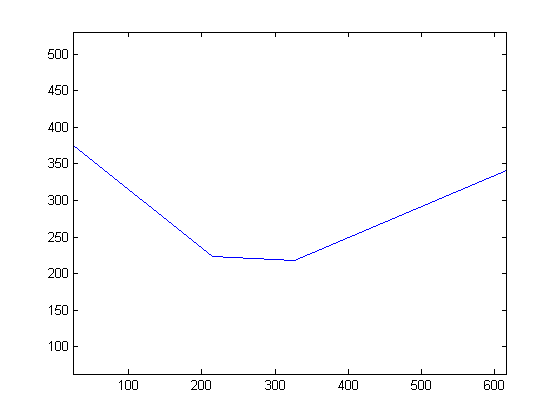This has three connected line segments. In this example, each segment has a different length. I wanted to be able to find a certain number of points that were equally spaced along this path.

#### IMPROFILE

After pondering this problem for a minute or so, I remembered that the function improfile does exactly this computation internally. The function improfile is used to compute pixel-value profiles along a user-specified polyline.

Let me illustrate improfile using a range image. In a range image, the pixel values represent estimated distance from the imaging device. I found the USF (University of South Florida) Range Image Database online. Below is a range image I downloaded from the database. It was taken by the Vision Lab at USF using a "K2T structured light camera." (No, I don't know what that is.) The web site helpfully tells me that the image is stored in "rasterfile format," which is pretty dated but fortunately supported by imread.

url = 'https://blogs.mathworks.com/images/steve/2012/chessmen.range';
imshow(I, 'InitialMagnification', 50)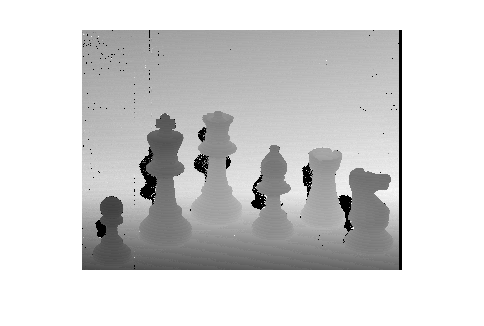Let's use improfile to compute a cross-section of range values along a particular path. First, though, let me superimpose the path on the image so you can where it is. (Although improfile has an interactive mode that lets you select the path using a mouse, I can't show that here.)

hold on
plot(x,y,'r','LineWidth',5)
hold off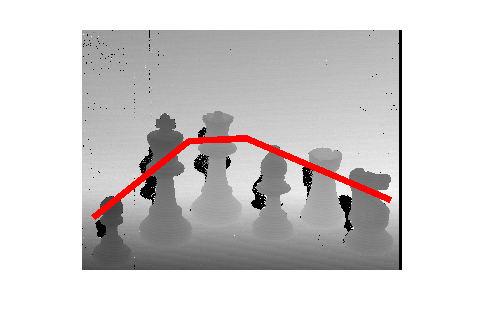Here's the cross-section of range values.

improfile(I,x,y)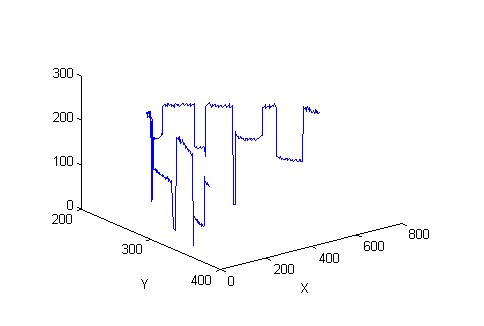You can see that improfile shows the cross-section as a 3-D plot. To see the cross-section as a normal 2-D line plot, call improfile with an output argument to get the cross-section values and then pass them to plot.

c = improfile(I,x,y);
plot(c)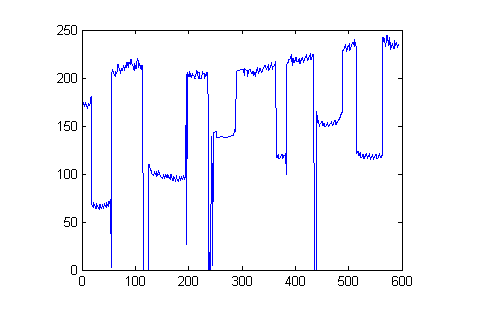#### Computing equidistant steps along the polyline

As part of its computation, improfile needs to compute equidistant steps along the polyline path. So how does that work?

The function first computes the cumulative distance from the beginning of the polyline to each vertex along the way, and then it uses a clever call to interp1 to compute the steps.

xy = [x' y'];
d = diff(xy,1)

d =

190  -152
113    -5
290   123


dist_from_vertex_to_vertex = hypot(d(:,1), d(:,2))

dist_from_vertex_to_vertex =

243.3187
113.1106
315.0063


cumulative_dist_along_path = [0;
cumsum(dist_from_vertex_to_vertex,1)]

cumulative_dist_along_path =

0
243.3187
356.4293
671.4356



Now we can get our steps by constructing a call to interp1.

num_points = 20;
dist_steps = linspace(0, cumulative_dist_along_path(end), num_points);
points = interp1(cumulative_dist_along_path, xy, dist_steps)

points =

24.0000  375.0000
51.5949  352.9241
79.1898  330.8482
106.7847  308.7722
134.3796  286.6963
161.9745  264.6204
189.5694  242.5445
218.0483  222.8209
253.3525  221.2587
288.6567  219.6966
323.9609  218.1345
356.7328  230.6108
389.2662  244.4095
421.7996  258.2081
454.3330  272.0068
486.8664  285.8054
519.3998  299.6041
551.9332  313.4027
584.4666  327.2014
617.0000  341.0000



Plot the points, superimposing them on the original path, to see how we did.

plot(x,y)
hold on
plot(points(:,1), points(:,2), 'o')
hold off
axis equal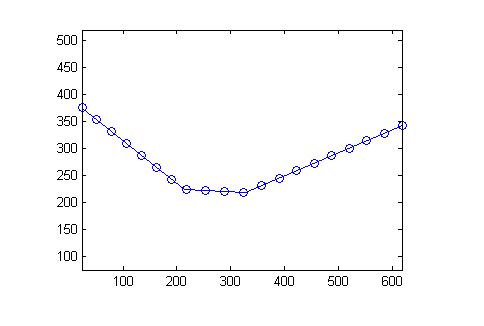There you go. Now you know about improfile, you know how to compute a path along a polyline, and you know about an online database of sample range imagery.

I think we should call it a day.

Published with MATLAB® 7.14

|